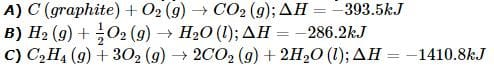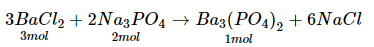# Test: Chemistry Mock Test - 10

## 45 Questions MCQ Test NEET Mock Test Series | Test: Chemistry Mock Test - 10

Description
Attempt Test: Chemistry Mock Test - 10 | 45 questions in 45 minutes | Mock test for NEET preparation | Free important questions MCQ to study NEET Mock Test Series for NEET Exam | Download free PDF with solutions
QUESTION: 1

Solution:
QUESTION: 2

Solution:
QUESTION: 3

### Methyl benzene can be prepared by reacting benzene with bromo-methane in the presence of

Solution:
QUESTION: 4
Which of the following sets of quantum numbers belongs to highest energy?
Solution:
QUESTION: 5
Acetaldehyde is the rearrangement product of
Solution:
QUESTION: 6
Night blindness is caused by the deficiency of
Solution:
QUESTION: 7
BCl₃ molecule is planar while NCl₃ is pyramidal because
Solution:
QUESTION: 8
Internal energy change when system goes from state A to B is 40 KJ/mol. If the system goes from A to B by reversible path and returns to state A by irreversible path, what would be net change in internal energy ?
Solution:
QUESTION: 9
Which one of the following informations can be obtained on the basis of Le Chatelier principle ?
Solution:
QUESTION: 10
In the titration between oxalic acid and acidified potassium permanganate, the manganous salt formed catalyses the reaction. The manganous salt is
Solution:
QUESTION: 11
One of the oxidants used with liquid propellants is
Solution:
QUESTION: 12

Calculate enthalpy of formation of ethylene from the following data:Solution:
QUESTION: 13

Which of the following has sp2 hybridisation ?

Solution:
QUESTION: 14
Which one of the following represents the correct order of electronegativity
Solution: The electronegativity values for the representative elements increase along a period from left to right and decrease down a group. This is because, as we move along the period from left to right nuclear charge increases and atomic radius decreases whereas when we move down the group, the atomic radius as well as screening effect increases. Thus, the order of electronegativity of N, O and P is
$O>N>P$
QUESTION: 15
Potassium permanganate oxidises sulphur dioxide to
Solution:
QUESTION: 16
Which of the following is not considered as an organometallic compound?
Solution:
QUESTION: 17
Cryolite is
Solution:
QUESTION: 18
Three faradays of electricity was passed through an aqueous solution of Iron (II) bromide. The weight of iron metal (at. wt = 50) deposited at the cathode is
Solution:
QUESTION: 19

If 0.44 g of a colourless oxide of nitrogen occupies 224 ml at 1520 mm hg and 273oC, then the compound is

Solution:
QUESTION: 20
The gas evolved on heating Na₂CO₃ is
Solution:
QUESTION: 21
Which one of the following elements is a non-metal
Solution:
QUESTION: 22

The ion that can be preciitated by HCl as well as H₂S is

Solution:
QUESTION: 23
Which one of the following oxides is most acidic ?
Solution:
QUESTION: 24
Copper turning when heated with conc.H₂SO₄ gives
Solution:
QUESTION: 25

Which of the following can give iodometric titration?

Solution:
QUESTION: 26

Alkaline earth metals form ions of the formula

Solution:
QUESTION: 27

Which of the following isomers will have the highest boiling point ?

Solution:
QUESTION: 28
The property of hydrogen which distinguishes it from alkali metals is
Solution:
QUESTION: 29
When ethylamine is heated with chloroform and alcoholic KOH, a compound with offensive smell is obtained. This compound is
Solution:
QUESTION: 30
pH of a solution is defined by the expression
Solution:
QUESTION: 31
Which of the following is not metamer?
Solution:
QUESTION: 32

Which of the following is least basic?

Solution:
QUESTION: 33
If acetyl chloride is reduced in the presence of BaSO₄ and Pd, then
Solution:
QUESTION: 34

following step is :
CH3 CH3 + Cl· → CH3 CH2 + HCl

Solution:
QUESTION: 35

Name the catalyst used in commercial method of preparation of phenol.

Solution:
QUESTION: 36
WHich of the following polymer is an example of fibre?
Solution:
QUESTION: 37
The electronegativity follows the order
Solution: The electronegativity values for the elements decrease down a group. This is because as we move down the group atomic radius as well as screening effect increases. Thus, the force of attraction with which the nucleus pulls the valence electrons towards itself decreases and consequently the electronegativity values also decrease. The electronegativity values follows the order:
F > Cl > Br > I
QUESTION: 38
A hydrocarbon has C = 85.72% and rest being hydrogen.The hydrocarbon is
Solution:
QUESTION: 39

The oxidation number of S in SO₄⁻2 is

Solution:
QUESTION: 40
To get n-type doped semiconductor, impurity to be added to silicon should have the following number of valence electrons
Solution:
QUESTION: 41
Which of the following is not a colligative property?
Solution:
QUESTION: 42
The number of asymmetric carbon atoms in tartaric acid is:
Solution:
QUESTION: 43

If 0.5 mol of BaCl₂ is mixed with 0.2 mol of Na₃PO₄, the maximum number of mole of Ba₃(PO₄)₂ that can be formed is

Solution:2 mol of Na3PO4 reacts with 3 mol of BaCl2 to form 1 mol Ba3(PO4)2
∴ 0.2 mol of Na3PO4 reacts with 0.3 mol of BaCl2 to form 0.1 mol Ba3(PO4)2.
Thus,Na3PO4 is limiting reagent here.

QUESTION: 44
Colloidal gold is prepared by
Solution:
QUESTION: 45
Which of the following is not a property of the transition elements?
Solution:Use Code STAYHOME200 and get INR 200 additional OFF Use Coupon Code

### How to Prepare for NEET

Read our guide to prepare for NEET which is created by Toppers & the best Teachers